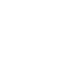So far in my previous blog post, I talked about the history of the Alfa Romeo (916) GTV.

I also talked about my intention to convert into an electric vehicle.

In this blog post we will look at the various types of electric motors commonly used in electric vehicle conversions.

## DC or AC Electric Motors?

Lets first understand what the heck I am talking about.

DC stands for Direct Current, whereas AC stands for Alternating Current.

In Direct Current, the positive (+) terminal is always positive, and the negative (-) terminal is always negative.

With AC Current, in a two wire system (known as a ‘Single Phase’ supply system), the electricity alternates, so that sometimes one wire has a positive voltage, and sometimes the ‘other’ wire instead.

The rate at which the voltage changes fully between the two wires, is known as the Frequency, and is measured in Hertz (Hz).

Notice I used the word ‘fully’, as the voltage does not instantly change from one wire to the other, but changes over a very short time period.

If you were to measure an AC voltage using a multimeter set to measure AC voltage, you would measure the RMS.

RMS is short for Root Mean Square, and is 0.707 of the peak voltage that occurs during a alternating cycle.

So if ‘wire A’ had a peak voltage of 100 volts AC, then the volt meter would read 0.707 of 100 volts, and therefore 70.7 volts AC.

It is necessary to measure the RMS figure because unlike DC, AC voltage is constantly changing (Alternating, hence the name Alternating Current).Single and Three Phase AC Motors

So now you hopefully understand what AC electricity is, lets look at the two main types of AC motor supplies you will come across.

Firstly ‘Single Phase’ is the two wire AC supply system that I described previously.

It is called Single Phase because it has a ‘phase wire’ which is often called the ‘live’ by the public.

Of course it has a second wire, known as the Neutral also.

Three Phase Supply systems have three Phase wires.

To understand better, first consider the Single phase AC system. The electricity is represented as a single sine wave, which goes up and down, above and below to zero volts point, over a period of time (picture to follow soon).

By contrast, a Three Phase AC system has three Sine waves, representing the three individual phases.

These three phases are 120 degrees apart (3 x 120 = 360 degrees), and therefore ‘peak voltage’ of each one occurs in sequence, at different time periods.

Three phase systems are the type that you will most likely be using in an electric vehicle build, as bigger more powerful motors, use three phase supplies.

DC Motors… to be continued, check back soon.

1.